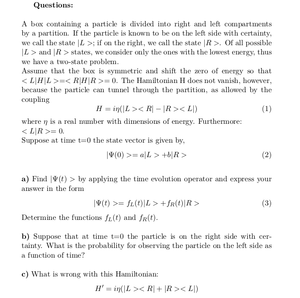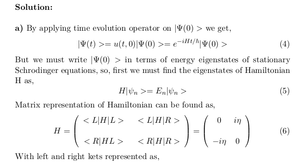Solved Problems in Physics, Physical Chemistry, and Computer Simulation Projects for College and Graduate Students.# Time Evolution of a Particle in a Box Divided into Right and Left Compartments

Regular price
\$6.90
Sale price
\$6.90
Tax included.

Questions:

A box containing a particle is divided into right and left compartments by a partition. If the particle is known to be on the left side with certainty, we call the state |L >; if on the right, we call the state |R >. Of all possible |L > and |R > states, we consider only the ones with the lowest energy, thus we have a two-state problem.

Assume that the box is symmetric and shift the zero of energy so that < L|H|L >=< R|H|R >= 0. The Hamiltonian H does not vanish, however, because the particle can tunnel through the partition, as allowed by the coupling

H = iη(|L >< R| − |R >< L|)            (1)

where η is a real number with dimensions of energy. Furthermore: < L|R >= 0.

Suppose at time t=0 the state vector is given by,

|Ψ(0) >= a|L > +b|R >                   (2)

a) Find |Ψ(t) > by applying the time evolution operator and express your answer in the form

|Ψ(t) >= fL(t)|L > +fR(t)|R >       (3)

Determine the functions fL(t) and f fR(t).

b) Suppose that at time t=0 the particle is on the right side with certainty. What is the probability for observing the particle on the left side as a function of time?

c) What is wrong with this Hamiltonian:

H' = iη(|L >< R| + |R >< L|)

By purchasing this product, you will get the step by step solution of the above problem in pdf format and the corresponding latex file where you can edit the solution. I am also available to help  you with any possible question you may have.

If you have any question about this solution, please send it to info@solutions4students.com

I'm available to help students with their homework assignments and computer simulation projects.